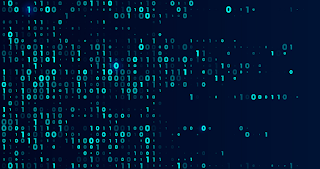# Exploring the Basics of Binary Code: Understanding the Language of ComputersExploring the Basics of Binary Code: Understanding the Language of Computers

Binary code is a system of encoding information using only two digits – 0 and 1. It is the most basic form of digital communication and is used extensively in computer programming, telecommunications, and digital electronics. In this article, we will take a closer look at binary code, its history, how it works, and its importance in the digital age.

## History of Binary Code

The concept of binary code dates back to the early 17th century, when the German mathematician and philosopher, Gottfried Wilhelm Leibniz, proposed the idea of a binary number system. He believed that all mathematics could be reduced to a series of 0s and 1s, and that this system could be used to represent all information in the universe. However, it wasn't until the advent of electronic computers in the 20th century that binary code became widely used.

## How Binary Code Works

Binary code works by representing information using a series of 0s and 1s. Each digit in the binary code is called a bit, and a group of eight bits is called a byte. In a computer system, these bytes are used to represent everything from letters and numbers to images and sounds.

Each binary digit represents a power of 2. The rightmost digit represents 2^0, the second digit from the right represents 2^1, the third digit represents 2^2, and so on. To convert a decimal number to binary, you divide the number by 2 and write down the remainder. You then divide the quotient by 2 and write down the remainder, and so on, until you reach a quotient of 0.

For example, the decimal number 15 can be converted to binary as follows:

15 ÷ 2 = 7, remainder 1 7 ÷ 2 = 3, remainder 1 3 ÷ 2 = 1, remainder 1 1 ÷ 2 = 0, remainder 1

Therefore, the binary representation of 15 is 1111.

## Importance of Binary Code in the Digital Age

Binary code is an essential component of the digital age, and it is used extensively in computer programming, telecommunications, and digital electronics. Without binary code, computers would not be able to store, process, and transmit information.

In computer programming, binary code is used to represent all data and instructions that are processed by the computer's central processing unit (CPU). This includes everything from basic arithmetic operations to complex algorithms.

In telecommunications, binary code is used to encode and transmit digital signals over long distances. This allows for the efficient and reliable transmission of data over networks, such as the internet and mobile networks.

In digital electronics, binary code is used to represent all information that is stored in digital memory. This includes everything from text and images to video and audio.

## Conclusion

Binary code is the language of computers and is an essential component of the digital age. It is a system of encoding information using only two digits – 0 and 1 – and is used extensively in computer programming, telecommunications, and digital electronics. Understanding binary code is essential for anyone who wants to work with computers or pursue a career in technology. It is the foundation of all modern computing and is essential for the development of new technologies that will shape the future.